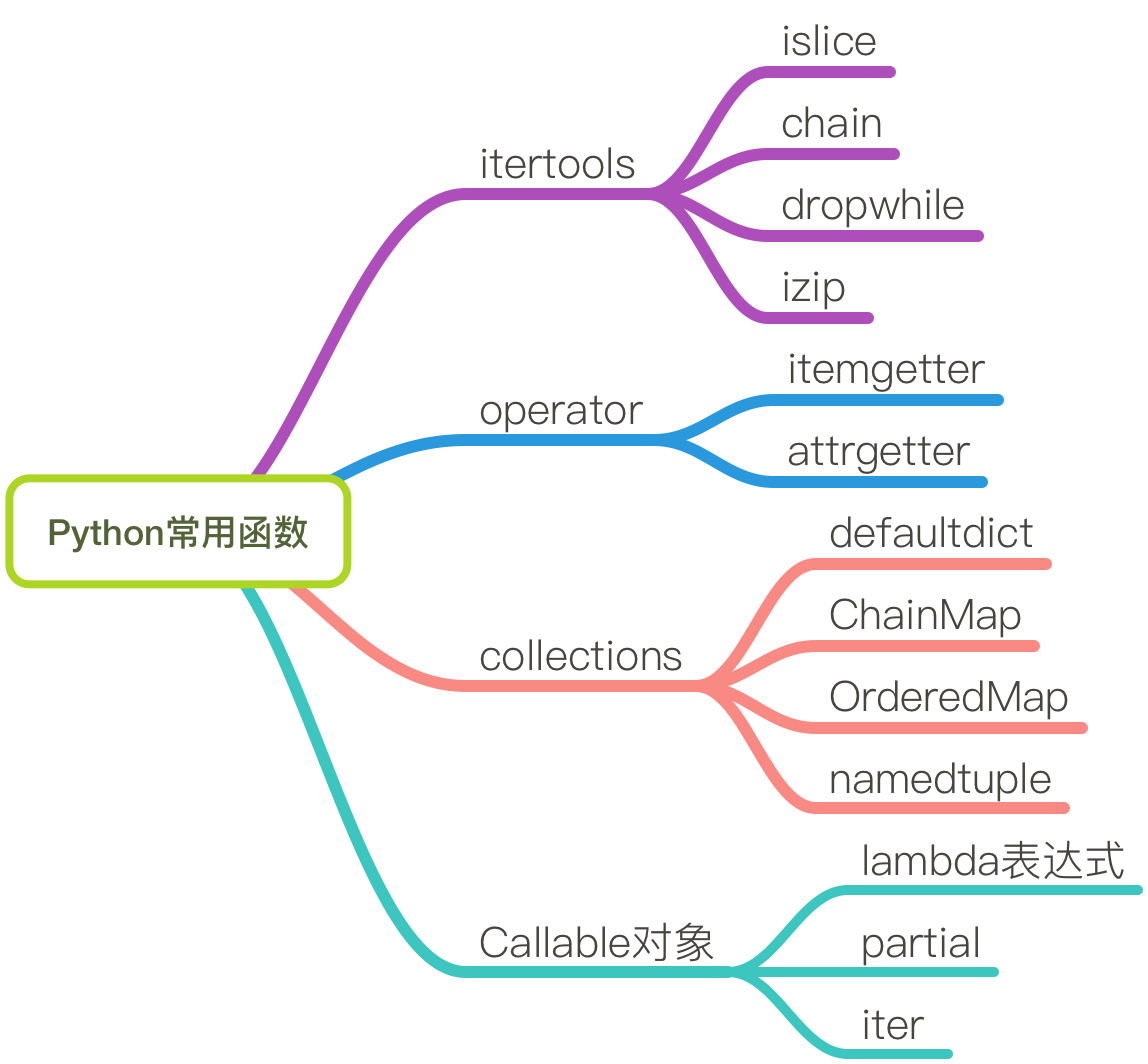# Python 常用模块记录

Posted by YuanBao on April 19, 2016## itertools – 控制迭代的模块

• chain(iter1, iter2, ...)：将多个迭代器连接起来，生成一个大的迭代器（使用 yield from 也可以实现类似的功能）
• dropwhile(callable, iter)：该函数从迭代器 iter 头反复调用 callable 函数，丢弃掉所有满足条件的元素，直到遇到第一个不满足条件的元素。

from itertools import dropwhile

with open('/etc/passwd') as f:
for line in dropwhile(lambda line: line.startswith('##'), f):
print(line, end='')


patterns = ["xxb", "xxc", "cbc", "xxd", "xxe"]

res1 = [p for p in patterns if not p.startswith("xx")]
res2 = list(dropwhile(lambda p: p.startswith("xx"), patterns))

#########################
res1 = ['cbc']
res2 = ['cbc', 'xxd', 'xxe']

• islice：给迭代器赋予切片的功能。正常情况下，迭代器是不能进行切片操作的，但是通过使用 islice 可以实现迭代器的切片操作。例如：
from itertools import islice

def range_iter(begin, end):
if begin < end:
yield begin
begin += 1

items = range_iter(0, 10)

for x in islice(items, 3, None):
print(x)


• izip 以及 longest_izip：针对迭代器实现 zip 的功能和 longest_zip 的功能。这个很好理解，不多说。

## operator 模块

operator 模块中的函数和类可以轻松地返回一个 callable 对象供我们使用，这在某些需要排序的场景中应用非常方便。

• attrgetter：该函数将属性名封装成为一个 callable 对象，该 callable 对象可以在类的实例上直接调用，并返回相应属性的值。看下面的例子：
from operator import attrgetter

class User:
def __init__(self, name, mail):
self.name = name
self.mail = mail

user = User("wky", "abc@gmail.com")
f = attrgetter(("name", "mail"))

print f(user)  # (user.name, user.mail)



attrgetter 返回的 callable 对象在很多时候都会极大地方便我们编码。例如我们有一个 User 实例的列表，我们想以 User 实例中的 name 属性对列表进行排序，我们可以这么写：

from operator import attrgetter
sorted(users, key=attrgetter('name'))

• itemgetter：同 attrgetter 类似，该函数可以将一个 key 封装成为一个 callable 对象，该对象可以在任何支持 __getitem__ 的实例上（例如字典）调用。这两个函数都在排序不支持原生比较的对象的应用场景中使用非常广泛。

## collections 模块

collections 模块为我们定义了很多易用的数据结构，当我们需要某个复杂但是常见的数据结构时，最好可以去 collections 模块中看一下。

• defaultdict：有时候我们需要可以把一个键映射到多个值上的字典，这时候我么就可以使用 defaultdict 了（当然你也可以自己实现）。需要注意的是，defaultdict 会默认为每个 key 初始化值，所以你只需要关注元素添加操作，例如：
from collections import defaultdict

d = defaultdict(list)
d['a'].append(1)  #自动创建键值'a'对应的列表
d['a'].append(2)
d['b'].append(4)

d = defaultdict(set)

• ChainMap：如果你想要把多个字典连接成为一个大的字典，那么 ChainMap 是你最好的选择：
a = {'x': 1, 'z': 3 }
b = {'y': 2, 'z': 4 }

from collections import ChainMap
c = ChainMap(a,b)
print(c['x']) # Outputs 1 (from a)
print(c['y']) # Outputs 2 (from b)
print(c['z']) # Outputs 3 (from a)


a = {'x': 1, 'z': 3 }
b = {'y': 2, 'z': 4 }
merged = dict(b)
merged.update(a)


• namedtuple：通过使用一个普通的元组对象来实现针对一个序列的命名，这将极大提高序列 操纵的可读性。

from collections import namedtuple

User = namedtuple('User', ["username", "gender", "phone"])

user = User(*res)



## callable 对象

#### lambda 表达式

add = lambda x, y: x + y


x = 10
a = lambda y: x+y
x = 20
b = lambda y: x+y

print a(10)  ## 结果是30
print b(10)  ## 结果也是30


x = 10
a = lambda y, x=x: x+y
x = 20

print a(10)  ##结果是20


#### partial

partial 在 Python 中是一个非常实用的函数。有很多的场景下，看似很难完成的工作都可以使用 partial 来完成。这个函数能够将某个函数和它的一部分参数进行打包，然后返回一个 callable 对象。我们只需要提供剩余的参数，既可以成功完成函数调用。因此，partial 最直观的功能就是减少函数的调用参数

points = [ (1, 2), (3, 4), (5, 6), (7, 8) ]

import math
def distance(p1, p2):
x1, y1 = p1
x2, y2 = p2
return math.hypot(x2 - x1, y2 - y1)


points.sort(key=partial(distance,(2,3)))


#### iter

def reader(s):
while True:
data = s.recv(1024)
if data == b'':
break
process_data(data)


def reader2(s):
for data in iter(lambda: s.recv(CHUNKSIZE), b''):
process_data(data)